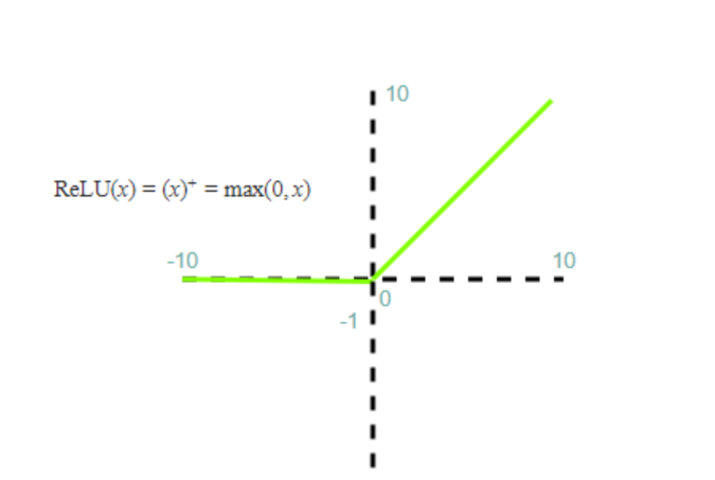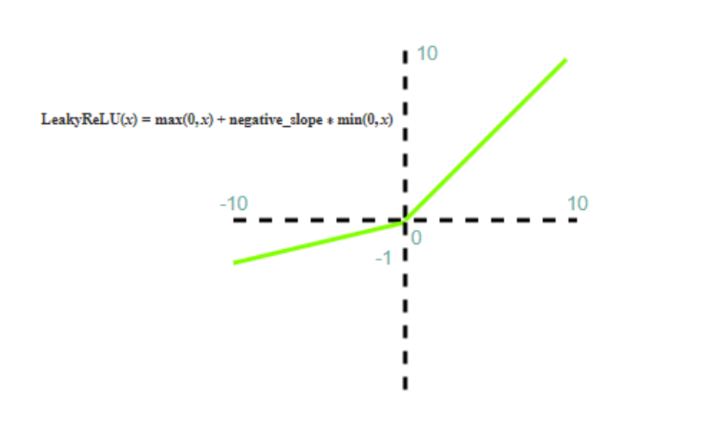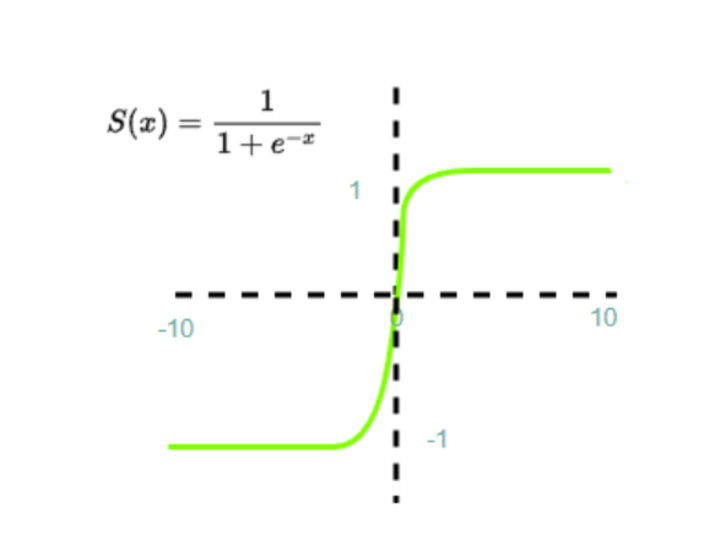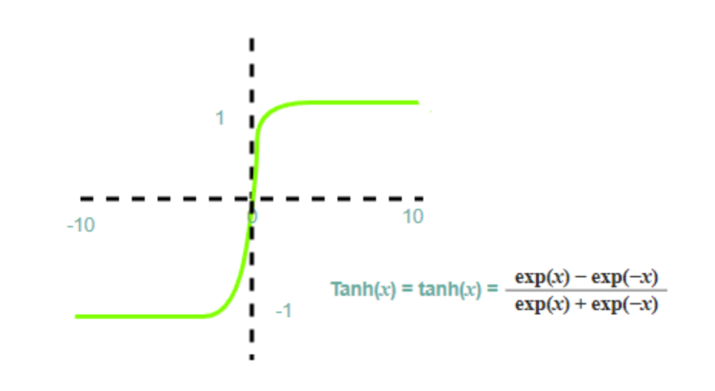# Activation Functions in Pytorch

Pytorch is an open-source machine learning framework that is widely used to create machine learning models and provides various functions to create neural networks. The activation function is a key component of neural networks. The activation function determines the output of a node in the neural network given an input or set of inputs to the node. The activation function introduces non-linearity in the output of a node of the neural network which is necessary for solving complex machine-learning problems.

## What is an Activation Function ?

Neural networks in artificial intelligence consist of an input layer, a hidden layer, and an output layer. The input layer takes the user input in different forms and the hidden layer performs some hidden calculations and the output layer gives the result which acts as the input for the next connected nodes. So the activation function is applied to the user input to perform the calculation and give the output through the output layer.

## Types of Activation Function Provided by Pytorch

Pytorch provides different activation functions that can be used in case of different machine learning problems.

• ReLU Activation Function

• Leaky ReLU Activation Function

• Sigmoid Activation Function

• Tanh Activation Function

• Softmax Activation Function

## ReLU Activation Function

Rectified linear activation function (ReLU) is a widely used activation function in neural networks. The ReLU function is defined as f(x) = max(0,x). It is a nonlinear function that always gives output as Zero for negative inputs and does not change the output for positive inputs. So, No learning takes place when input is negative.ReLU function can be imported from the torch.nn package. The graph of the ReLU function is shown below −### Example

In the below example we created an array and passed it through the Relu activation function. The Relu activation function converts each input element of the array according to the Relu activation function.

#pip install numpy
import numpy as np

def relu(x):
return np.maximum(0, x)

x = np.array([-1, 2, -3, 4, 0])
y = relu(x)

print(y)


### Output

[0 2 0 4 0]


## Leaky ReLU Activation Function

Leaky ReLU Activation function is similar to ReLU activation function but Leaky ReLU function does not give zero output for negative inputs so there is learning in case of negative inputs also. The leaky ReLU function is defined as LeakyReLU(x)=max(0,x)+negative_slope∗min(0,x). The graph of the Leaky ReLU function is shown below.Leaky ReLU is also non-Linear and solves the problem of dying neurons which occurs when the function gives zero output or no learning/ training of neurons in case of negative input values.

### Example

In the below example we created an array using numpy and passed the array through the leaky_relu function which converts each element of the array according to the leaky_relu function.

import numpy as np

def leaky_relu(x, alpha=0.1):
return np.maximum(alpha * x, x)

x = np.array([-1, 2, -3, 4, 0])
y = leaky_relu(x)

print(y)


### Output

[-0.1  2.  -0.3  4.   0. ]


## Sigmoid Activation Function

The sigmoid function is one of the most commonly used activation functions in neural networks. It is defined as f(x) = 1/(1+exp(-x)). The sigmoid function maps any input to a value between 0 and 1. It is useful for binary classification problems where the output is either 0 or 1. The sigmoid function is also used in deep neural networks as an activation function for the output layer. The output of the sigmoid function is often considered a probability in binary classification problems. The graphical representation of the sigmoid Activation function is shown below.Vanishing gradient problems can occur in sigmoid function.

### Example

In the below example we created an array using numpy and passed the array through the sigmoid function which converts each element of the array according to the sigmoid function.

import numpy as np

def sigmoid(x):
return 1 / (1 + np.exp(-x))

x = np.array([-1, 2, -3, 4, 0])
y = sigmoid(x)

print(y)


### Output

[0.26894142 0.88079708 0.04742587 0.98201379 0.5       ]


## Tanh Activation Function

Hyperbolic tangent function is a nonlinear activation function which gives an output between -1 and 1 for any input value.The tanh activation function is defined as f(x) = (exp(x)-exp(-x))/(exp(x)+exp(-x)). The S-shaped graph of the Tanh function is shown below.Vanishing gradient problem when using Tanh function.

### Example

In the below example we created an array using numpy and passed the array through the tanh function which converts each element of the array according to the tanh function.

import numpy as np
def tanh(x):
return np.tanh(x)
x = np.array([-1, 2, -3, 4, 0])
y = tanh(x)
print(y)


### Output

[-0.76159416  0.96402758 -0.99505475  0.9993293   0.        ]


## Softmax Activation Function

The softmax function is used for multi-class classification problems. It is defined as f(x) = exp(x_i)/sum(exp(x_j)) for all i. The softmax function maps any input to a probability distribution over the classes. It is useful for multi-class classification problems where the output can belong to one of several classes. The softmax function is also used in deep neural networks as an activation function for the output layer. The softmax function is generally used in combination with other activation functions and placed at the last layer.

### Example

In the below example we created an array using numpy and passed the array through the softmax function which converts each element of the array according to the softmax function.

import numpy as np
def softmax(x):
exp_x = np.exp(x - np.max(x)) # to prevent overflow
return exp_x / np.sum(exp_x)
x = np.array([1, 2, 3, 4, 5])
y = softmax(x)
print(y)


### Output

[0.01165623 0.03168492 0.08612854 0.23412166 0.63640865]


## Conclusion

Activation functions play a crucial role in neural networks, and PyTorch provides a wide range of activation functions to choose from. The choice of activation function depends on the type of problem and the structure of the neural network. By using the appropriate activation function, we can improve the performance of our neural network and achieve better results.

Updated on: 17-Apr-2023

526 Views